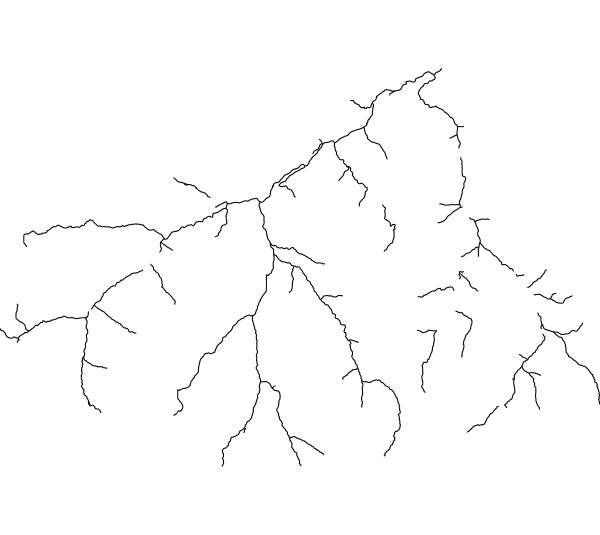## NAME

r.green.hydro.optimal - Detect the position of the potential hydropower plants that can produce the highest possible power

raster

## SYNOPSIS

r.green.hydro.optimal
r.green.hydro.optimal --help
r.green.hydro.optimal [-dc] elevation=name discharge=name river=name len_plant=float len_min=float distance=float [p_max=float] p_min=float output_plant=name [output_point=name] efficiency=float [--overwrite] [--help] [--verbose] [--quiet] [--ui]

### Flags:

-d
Debug with intermediate maps
-c
Clean vector lines
--overwrite
Allow output files to overwrite existing files
--help
Print usage summary
--verbose
Verbose module output
--quiet
Quiet module output
--ui
Force launching GUI dialog

### Parameters:

elevation=name [required]
Name of input elevation raster map
discharge=name [required]
Name of river discharge [m^3/s]
Name of input raster map
river=name [required]
Name of vector map with interested segments of rivers
Or data source for direct OGR access
len_plant=float [required]
Maximum length of the plant [m]
Default: 10000
len_min=float [required]
Minimum length of the plant [m]
Default: 10
distance=float [required]
Minimum distance among plants [m]
Default: 0.5
p_max=float
Maximum mean power [kW]
p_min=float [required]
Minimum mean power [kW]
Default: 10.0
output_plant=name [required]
Name of output vector map with potential power for each river segment [kW]
Name for output vector map
output_point=name
Name of output vector map with potential power intakes and restitution [kW]
Name for output vector map
efficiency=float [required]
Efficiency [-]
Default: 1

## DESCRIPTION

r.green.hydro.optimal detects the position of the potential hydropower plants that can produce the highest possible power. Deciding the range of plant length and the distance between plants, the module returns two vector maps with the segments of rivers exploited by the potential plants and with the intakes and restitution of these plants. The module computes the potential plants in order to maximize the power that can be produced.

## NOTES

The three input files are the rivers considered (vector), the discharge for each point of this river (raster) and the elevation raster map to calculate the gross head.

The power is defined as:
P=η * ρ * g * Q * Δh
where η is the efficiency of the plant
ρ the density of water (1000 kg/m3)
g the gravity term (9,81 m/s2)
Q the discharge of the river
Δh the gross head of the considered segment

The module maximizes the power over a given range by a brute-force search in order to examine all possible arrangements of Q and Δh. Thus, the potential segments can be shorter than the maximum plant length chosen because it depends on the maximization of the product Q * Δh.

For each potential segment, the potential power is given in kW in attribute.

## EXAMPLE

This example is based on the case-study of Gesso and Vermenagna valleys in the Natural Park of the Maritime Alps, Piedmont, Italy.

Here is the vector file availablestreams of the interested streams in which we want to compute the potential hydropower plants. The river segments already exploited by an existing plant do not appear in the file.Input vector map availablestreams

The following command computes the potential plants for a plant length range from 10 to 800 m and a distance between plants of 800 m :

```r.green.hydro.optimal
discharge=discharge
river=availablestreams
elevation=elevation
len_plant=800
distance=800
output_plant=potentialsegments
output_point=potentialpoints
d.vect map= potentialpoints color=red
d.vect map= potentialplants color=blue```

The output vector maps are shown in the following picture which gathers the potential segments vector map (potentialplants, in blue) and the potential intakes and restitution vector map (potentialpoints, in red)Output vector maps potentialplants (in blue) and potentialpoints (in red)

r.green.hydro.discharge
r.green.hydro.delplants
r.green.hydro.theoretical
r.green.hydro.recommended
r.green.hydro.structure
r.green.hydro.technical
r.green.hydro.financial

## AUTHORS

Giulia Garegnani (Eurac Research, Bolzano, Italy), Manual written by Julie Gros.

## SOURCE CODE

Available at: r.green.hydro.optimal source code (history)

Latest change: Monday Jan 30 19:52:26 2023 in commit: cac8d9d848299297977d1315b7e90cc3f7698730

© 2003-2023 GRASS Development Team, GRASS GIS 8.3.1dev Reference Manual